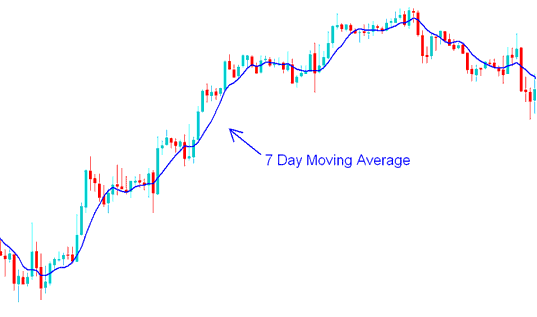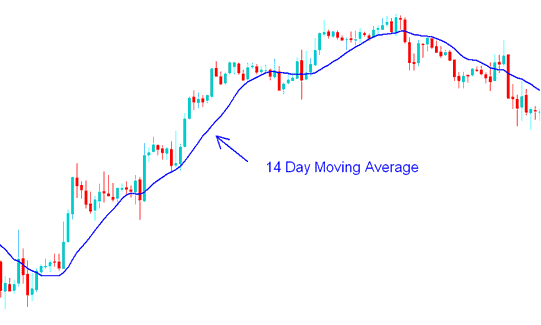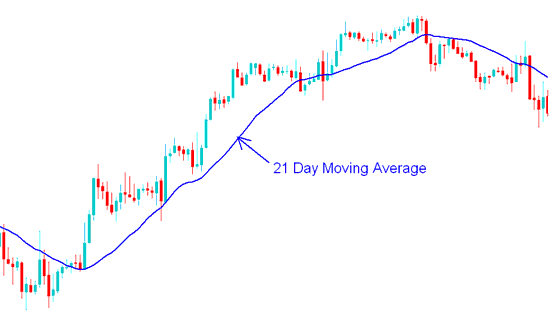# Trading Short Term and Long Term Forex Price Period of Moving Average

A forex trader can choose to adjust the forex price periods used to calculate the moving average.

If a forex trader uses short price periods then the MA will react faster to the changes in forex price.

For example if a forex trader uses the 7 day forex moving average then, the moving average indicator will react to the forex price change much faster than a 14 day or 21 day forex Moving Average would. However, using short time forex price periods to calculate the MA might result in the indicator giving false forex trading signals (whipsaws).7 Day Moving Average - Moving Average Forex Trading Strategies

If another trader uses longer time periods then the MA will react to price changes much slower.

For example, if a trader uses the 14 day MA then the average will be less prone to whipsaws but it will react much slower.14 Day Moving Average - Moving Average Forex Trading Strategy Example21 Day Moving Average - Moving Average Forex Trading Strategies Example